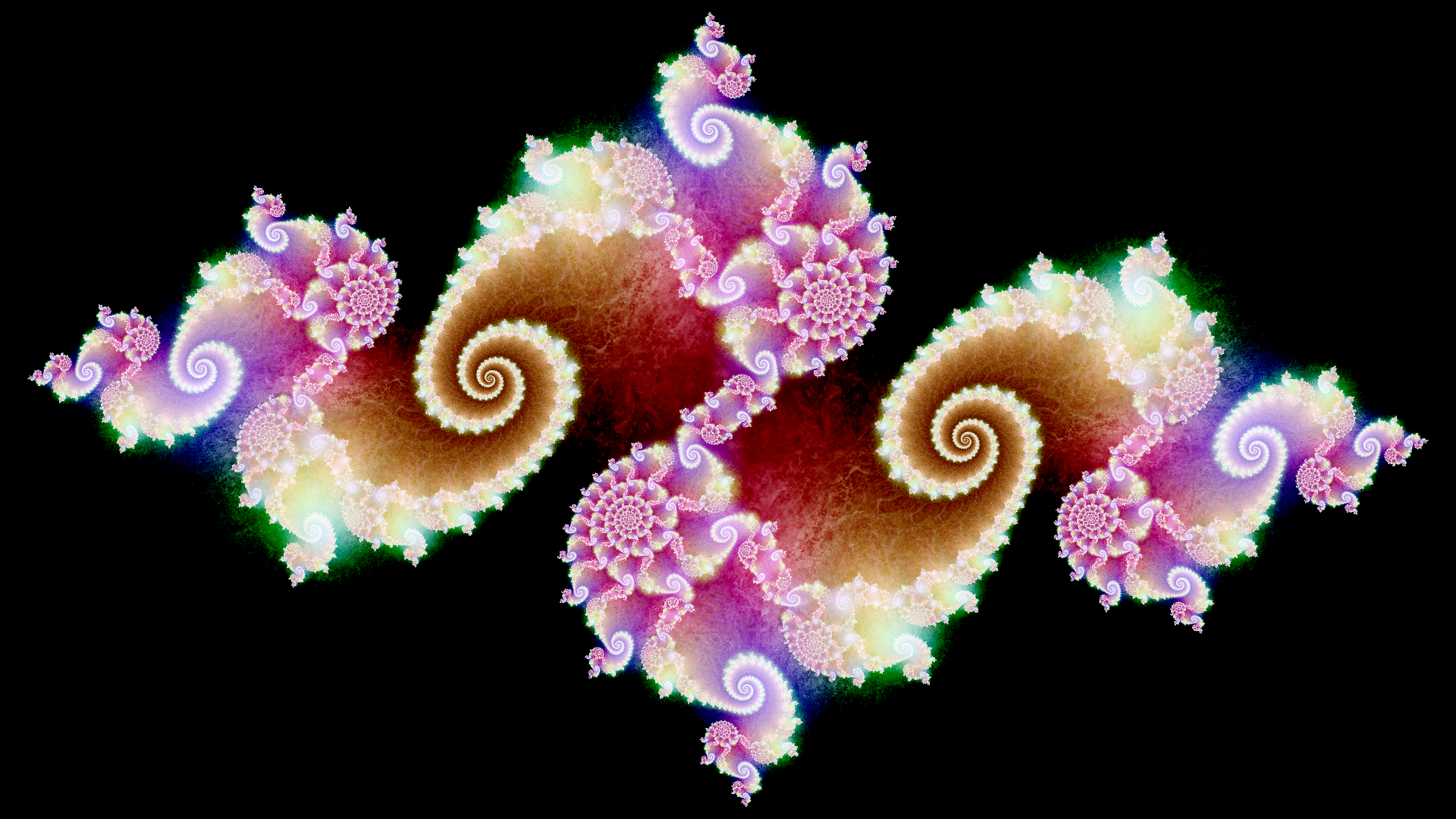# Folding the Julia Fractal

So the Julia sets are a class of sets that have became very popular because of their beauty and an interesting object of study. The definition of a Julia set is really simple, it is the set of complex numbers $$\bold S_c$$ such that for $$z \in \bold S_c$$, we have $$|f^n_c(z)| \le 2$$, where $$f_c(z) = z^2 + c$$. Each choice of $$c$$ gives rise to a different Julia set with distinct looks. I might write more about these stuff maybe but given how popular this subject is you can find a lot of it's cool properties anywhere on the internet. I suggest checking out Inigo Quilez on the subject of rendering them.

I hope your internet's good. Due to the nature of the subject it does require rather large images.

Given how simple the definitions of these sets are, it makes it really easy and straight-forward to render them. It can be done in a few lines and is one of the first things I ever coded.

The source code for the above is here, you can use the mouse to control the value of $$c$$. I left the shitty code there for sorta a historical record. Read with caution, it's painful to the eyes.

The procedure to render a Julia fractal is very simple:

1. Start with a complex number $$z$$
2. Successively compute $$z=z^2+c$$ for a maximum of $$N$$ iterations. A larger $$N$$ gives a more detailed fractal.
3. If $$|z|>2$$ at any iteration, prematurely stop the computation

For the above GIF, I simply assigned the number of iterations to reach step 3 as the colour: White if step 3 was never reached and different values of grey otherwise.

The creative part comes with how to shade it. I came across a method that involves procedural orbit traps here and attempted to replicate it.

The source code and demo is here. Instead of generating the cloud-like texture procedurally, a texture is used instead. It runs real time and hopefully on mobile browsers too so do give the link a click. The fractal above uses a value of $$c$$ around the neighbourhood of $$M_{23,2}$$, a Misiurewicz Point that gives the fractal a lacy appearance.

Speaking of lacy, another cool way to render is by estimating it's distance function. I did this a long time ago and probably deleted the source code along the way.

Given the seeming complexity of these fractals, I wondered if there was a way to visualize the formation of them. Since they form via very simple rules, there should be a nice way to depict them. My first attempt was to naively interpolate between each iteration, allowing me to create a smooth animation despite the otherwise discrete procedure. Trying many many ways to interpolate the best I got was this:

It looks cool but imo doesn't show how the very crucial $$z^2+c$$ plays a role in it's formation. That took me 2 hours by the way.

Next I had the idea of mapping. The operation $$f_c(z) = z^2+c$$ maps a point $$z$$ to a point $$z^2+c$$. The mapping that forms the Julia fractal $$f^n_c$$ is simply that mapping applied several times. Here's my attempt at visualizing $$f_c$$:

The first half of the GIF describes the $$+c$$ operation, which is simply a shift in the direction of $$c$$ . The second half describes the $$z^2$$ operation, which is sorta a 'squishing' of the space rotationally, resulting in 2 copies of the image. At the end of the first iteration, we have two displaced copies of the image:

Now, every iteration would generate $$\times 2$$ more copies, and you can see how the fractal gets more and more complicated at each iteration.

The above took forever to render by the way, given how slow python really is. Maybe 40fps is a little overkill for a gif but I digress.